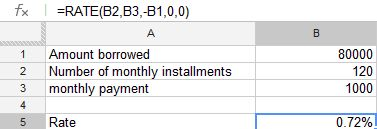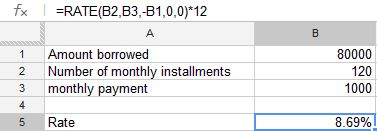## How much interest I can afford

The RATE function returns the interest rate of an investment, loan, or annuity based on a series of regular periodic cash flows.

The syntax of the rate function is

RATE(NPER, PMT, PV, FV, type, guess)

NPER:  The number of periods. NPER is a number value and must be greater than or equal to 0.

PMT: The payment made or amount received each period. payment is a number value. At each NPR, an amount received is a positive amount and an amount invested is a negative amount. It is simple monthly payment you are intended to make or receive

PV: (present value) The value of the initial investment, or the amount of the loan or annuity. present-value is a number value. At time 0, an amount received is a positive amount and an amount invested is a negative amount. In other words the amount of loan you intended to take

FV: An optional argument that represents the value of the investment or remaining cash value of the annuity (positive amount), or the remaining loan balance (negative amount), after the final payment. future-value is a number value. it could be ballon amount.

Type: Type (optional) defines whether the payment is due at the beginning (1) or the end (0) of a period

Guess: Guess (optional) determines the estimated value of the interest with iterative calculation. normally it is ignored

lets understand the function with an example:

I want to borrow 80,000 and make monthly payment for 10 years. The maximum monthly payment i can afford is 1,000. what is the maximum interest rate i can afford?

Solution:

In the above example

NPER: number of month here we have been provided with 10 year that means NPER is equal to 10 X 12 = 120

PMT: Payment for the period, in our example it is given as 1,000 per month

PV: present value. here the present value will be 80,000 which is amount i am borrowing now. we are inputting this value with – before it, because it will be cash outflow for the lender.

FV: it is an optional argument in out case we will put 0

Type: it can be either 1 or 0,  in our example we are putting 0 assuming the installment will be paid at the end of the period (i.e. month)

Guess: we can leave this argumentthe formula in cell B5 is =RATE(B2,B3,-B1,0,0)

I got the as 0.007241020100393 which i formatted using the % to convert it 0.72%

the above rate is period rate we have to convert this into annual rate

to convert this to annual rate we have multiplied it with 12for the formula in cell B5 is =RATE(B2,B3,-B1,0,0)*12

the solution to the problem is

i can afford up to 8.69% interest for my loan of 80,000/- for a period of 10 years

Advertisements